﻿ Fluidized bed (RANS) - XSim

Fluidized bed (RANS)

Update: July 28, 2017
OpenFOAM 4.x

Case directory

\$FOAM_TUTORIALS/multiphase/twoPhaseEulerFoam/RAS/fluidisedBed

Summary

We calculate the air flow blowing in from the bottom of air-mixed sand for 2 seconds. The calculation is performed as a two-dimensional problem with one mesh in the z-direction.Model geometry

The physical properties of air and particles are set in the files "constant/phaseProperties", "constant/thermophysicalProperties.air", "constant/thermophysicalProperties.particles".

The turbulence settings for air and particles are set in the files "constant/turbulenceProperties.air" and "constant/turbulenceProperties.particles". The standard k-ε model is used for air, and the kinetic model by B.G.M. van Wachem is used for particles.

The meshes are as follows, and the number of mesh is 6000.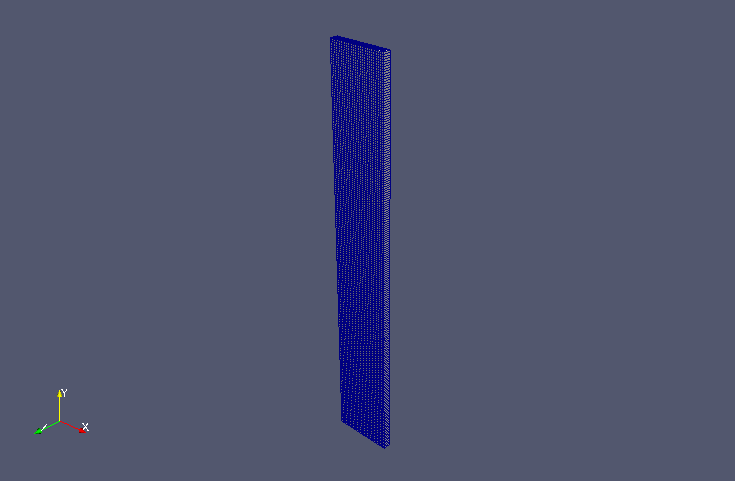Meshes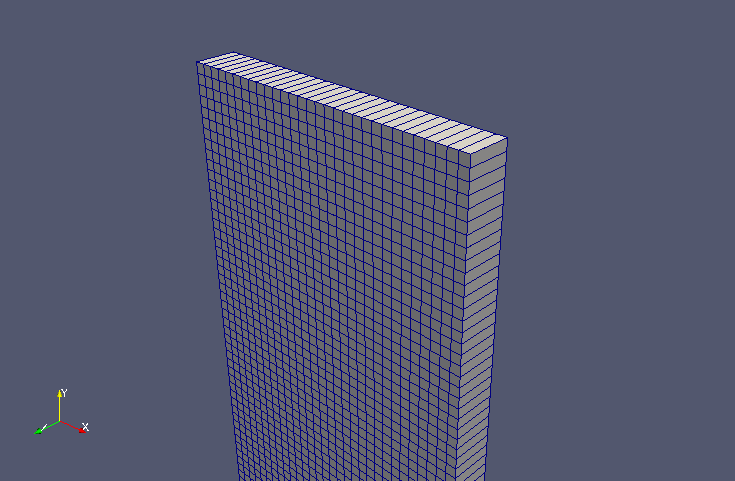Meshes (zoomed)

The calculation result is as follows.

Volume ratio of particles (alpha.particles)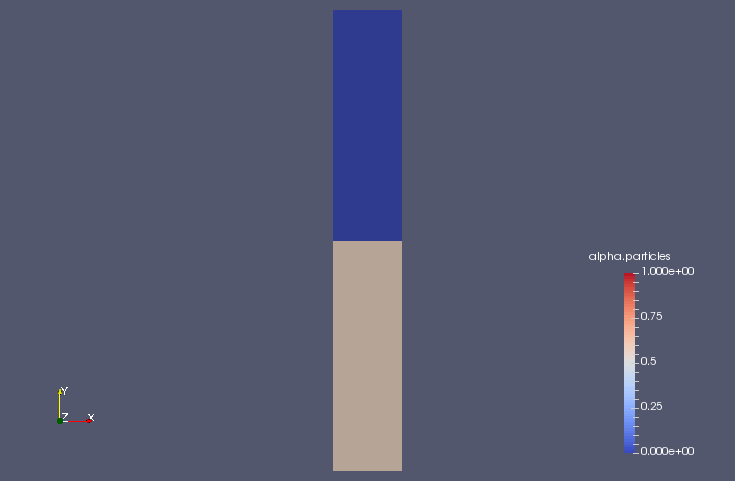Volume ratio of particles at initial time (alpha.particles)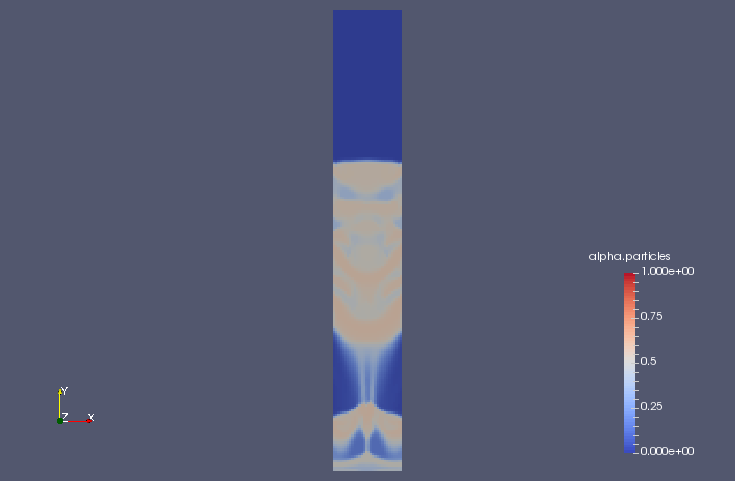Volume ratio of particles at 0.6 sec (alpha.particles)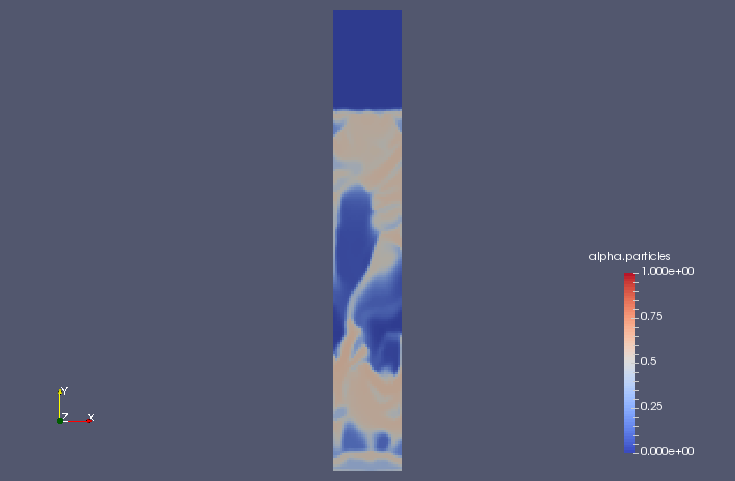Volume ratio of particles at 1 sec (alpha.particles)

Commands

cp -r \$FOAM_TUTORIALS/multiphase/twoPhaseEulerFoam/RAS/fluidisedBed fluidisedBed
cd fluidisedBed

blockMesh
setFields

twoPhaseEulerFoam

paraFoam

Calculation time

20 minutes 59.43 seconds *Single, Inter(R) Core(TM) i7-2600 CPU @ 3.40GHz 3.40GHz